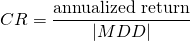# Calmar Ratio

The Calmar ratio (CR) is a performance measure that considers the drawdown of a fund to assess the fund’s performance. That is why the Calmar ratio is sometimes also referred to as the Drawdown ratio. The drawdown ratio was first proposed by Terry Young in the practitioners journal ‘Futures‘ in 1991. The Calmar ratio is mainly used to evaluate the peformance of Managed Futures funds or Commodity Trading Advisors (CTAs). Managed Futures funds are a type of trend-following hedge funds that invest in liquid futures contracts.

## Calmar Ratio Definition

The exact definition of the CR is provided by Young in the Futures magazine:

The Calmar ratio uses a slightly modified Sterling Ratio- average annual rate of return for the last 36 months divided by the maximum drawdown for the last 36 months – and calculates it on a monthly basis, instead of the Sterling ratio’s yearly basis.

It is clear from the definition that the ratio looks at return versus drawdown risk. Since the ratio uses smoothed values for the annual rate of return and the drawdown, the calmar is a smoothed version of the Sterling ratio. This has the advantage of smoothing out periods of overperformance and underperformance.

It is important to note that we do not subtract the risk-free rate from the annualized return. This is different from Sharpe ratio, where we do subtract the risk-free rate.

## Calmar ratio formula

The Calmar ratio (CR) formula is the followingBoth the annualized return and the maximum drawdown (MDD) are based on the last 36 months of monthly returns. To calculate the annualized return and the drawdown, see the page on how to annualize returns and how to calculate the drawdown. The maximum drawdown is the highest drawdown over the period considered.

Let’s take an example to illustrate the calculation of the ratio. Let’s assume we have a fund called Alpha Investments LLC. We retrieve the monthly net returns for the past 36 months. We then calculate the average monthly return, which turns out to be 1.5% per month. Annualizing this return, we obtain x%. Next, we also calculate the drawdowns over the past 36 months and take the highest value. Then we divide the annualized return by the MDD to obtain the CR ratio.

## What is a good Calmar Ratio?

Obviously, the higher the better. However, just like it is impossible the answer the question of what Sharpe ratio is good enough, the same applies to the Calmar ratio. One approach to answer the question is to look at what CR corresponds to a certain Sharpe ratio (under a normal distribution). This should give us some guidance of what values for the CR are realistic. The Excel spreadsheet at the bottom allows just that.

## Origin name

Finally, why is the Calmar Ratio called ‘Calmar’? The name is an acronym for the Young’s company name and newsletter: ‘CALifornia Managed Accounts Reports’.

## Summary

We discussed the Calmar ratio, a ratio that looks at the trade-off between return and downside risk. It is a measure that is often used to assess alternative investments.

### Drawdown ratio calculation

Want to implement the CR ratio? Download the Excel file that implements the CR: Calmar Ratio.